# How to Sum Entire Column or Row in Excel

No matter you are familiar or not familiar with excel functions, I think you must know SUM function, one of the most common used functions in excel. Generally, we often use SUM function to sum data in a fixed range in daily life. But if we want to sum entire column or row without boundary, how can we do? In fact, we can directly enter entire column or row as a range to apply SUM function. In this article, we will show you the method to sum entire column or row in excel, besides, we will also show you some simple examples to introduce and explain SUM function. Actually, in our daily work, we may meet problems which are more complex than these instances which only apply with simple formulas, anyway, you can use SUM function with other excel functions together to solve your problem.

EXAMPLE: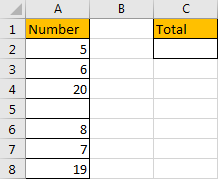This is a very simple example. As there is no criteria or reference, just to sum total amount for these numbers, we can use SUM function directly. Actually, there are three ways to sum data.

FORMULA 1:

Step 1: In C2, enter the formula =SUM(A2,A3,A4,A6,A7,A8).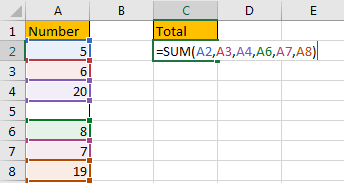Step 2: Press Enter to load result.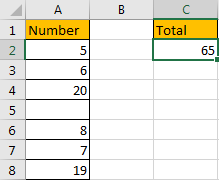HOW THIS FORMULA WORKS:

For SUM function, the syntax is:

=SUM(number1,[number2],…)

In this formula, for each argument, it can be a real number or a cell, or even a range. In this instance, this formula is easily to understand, we just enter cell which contains a number one by one into the formula. After pressing Enter, correct result is returned.

FORMULA 2:

As we mentioned above, for SUM function, the argument can be a number or a range, so we can enter a range instead of each real number into the formula. Let’s try again.

Step 1: In C2, enter the formula =SUM(A2:A8).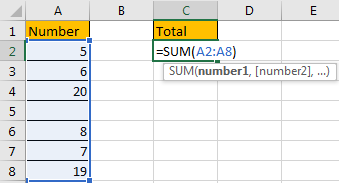Step 2: Press Enter and check result. We can get the same result.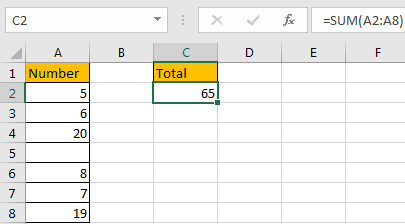HOW THIS FORMULA WORKS:

These numbers are included in range A2:A8, so we enter formula =SUM(A2:A8) here. In this formula, blank cell will be ignored automatically. We can also enter another range, just make sure the range contains all numbers. See screenshot below.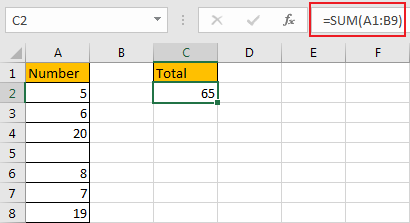FORMULA 3:

If your number list is long, and you don’t want to drag the scrollbar to the end to select the range, you can enter A:A into the formula directly.

Step 1: In C2 enter the formula =SUM(A:A).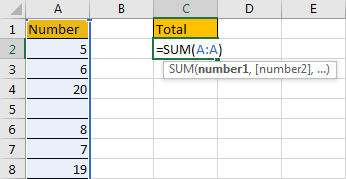Step 2: Press Enter and check result. We can get the same result.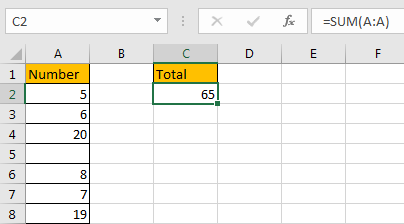HOW THIS FORMULA WORKS:

If you don’t know the bound of your number list or range, you can enter entire column into your formula, thus all numbers in the column are included, no data missing. You can also enter row number in the formula to sum data for an entire row.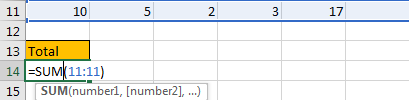The result is calculated correctly.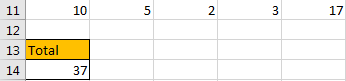Now we can know that:

SUM(A:A) means sum entire column (column A);

SUM(11:11) means sum entire row (row 11).

We can replace column and row with our own data in our daily life.

If data exists in two columns, just enter two ranges (A:A,B:B) in the formula. The first range is entire column A, so enter A:A, the second range is entire column B, so enter B:B.  See example below.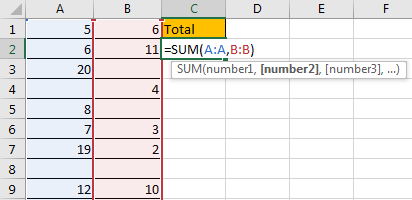After pressing Enter, we can get correct result.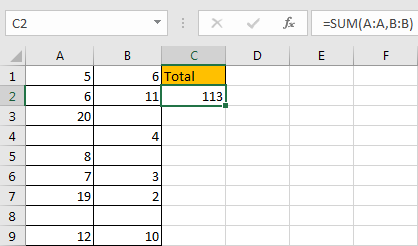NOTE:

Refer to above examples, we can enter columns or rows to sum data per our demand properly, but if cell is included in multiple ranges in SUM function, it will be calculated multiple times. See example below.

In formula =SUM(A:A,B:B,9:9), A9 and B9 are counted twice. So result in F2 is 22 greater than F7.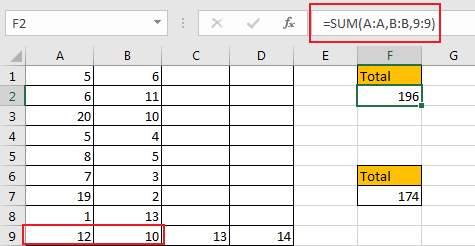But if you apply formula =SUM(A1:D9), there is only one range, so data will not be counted twice.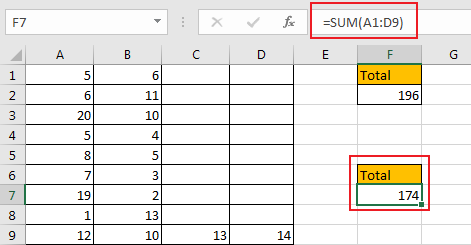### Related Functions

• Excel SUM function
The Excel SUM function will adds all numbers in a range of cells and returns the sum of these values. You can add individual values, cell references or ranges in excel.The syntax of the SUM function is as below:= SUM(number1,[number2],…)…